# NCERT Chemistry Class 12 Chapter 2 Intext Solutions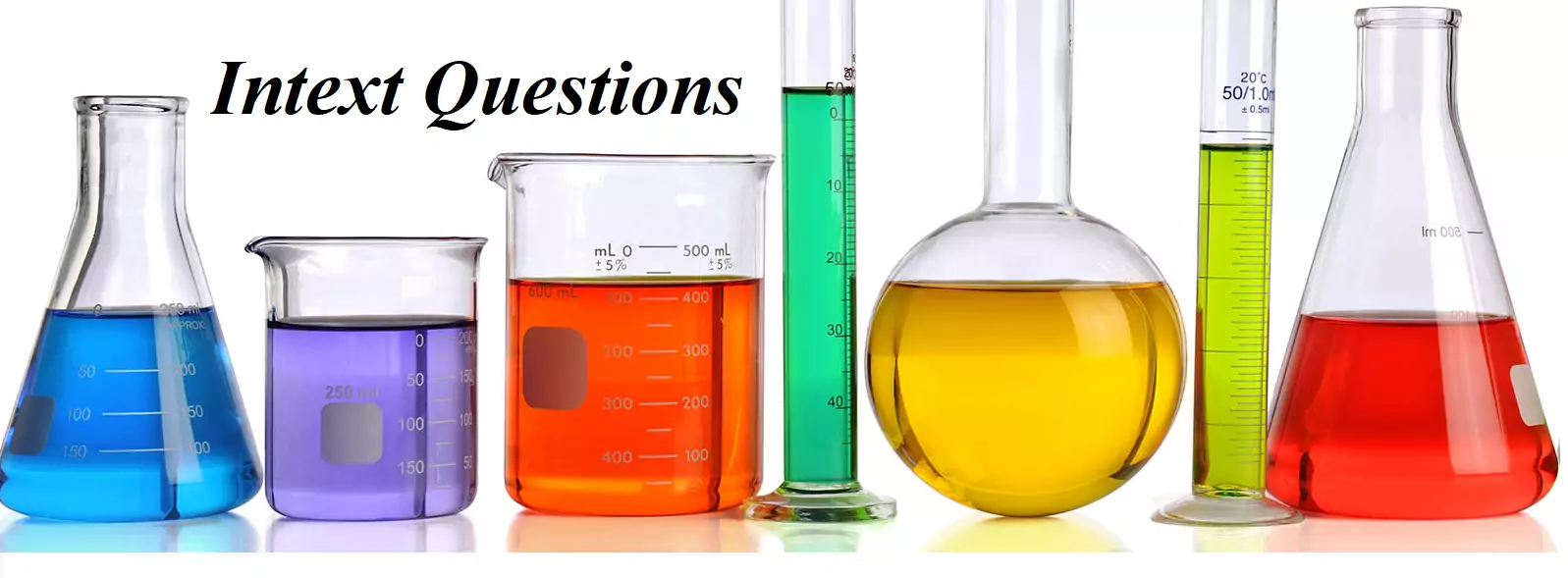NCERT Chemistry Class 12 Chapter 2 Intext Solutions

NCERT
Intext Questions
Chapter – Solutions

Que 1. Calculate the mass percentage of benzene (C6H6) and carbon tetrachloride (CCI4) if 22 g of benzene is dissolved in 122 g of carbon tetrachloride.
Ans 1. Mass of solution = Mass of Benzene (C6H6) + Mass of Carbon Tetrachloride (CCl4)
= 22 g +122 g = 144 g
Mass percentage of Benzene = 22/144 × 100 =15.28 %
Mass % of CCl4 = 122/144 × 100 = 84.72 %

Que 2. Calculate the mole fraction of benzene in solution containing 30% by mass in carbon tetrachloride.
Ans 2. Let the mass of Solution = 100 g
∴ Mass of Benzene in the solution = 30 g
∴ Mass of Carbon Tetrachloride = 100 – 30g = 70g
Molar mass of Benzene (C6H6) = 78 g mol-1
Molar mass of CCl4 = 12 + 4 × 35.5 = 154 g mol-1
∴ No. of moles of benzene = 30/78 mol = = 0.3846 mol
Molar mass of CCl4 = 1 × 12 + 4 × 35.5 = 154 g/mol
Therefore, Number of moles of CCl4 = 70/154 = 0.4545 mol
Thus, the mole fraction of C6H6 is given as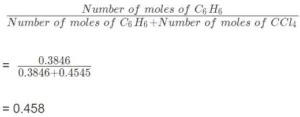NCERT Chemistry Class 12 Chapter 2 Intext Solutions

Que 3. Calculate the molarity of each of the following solutions
(a) 30 g of Co(NO3)26H2O in 4·3 L of solution
(b) 30 mL of 0-5 M H2SO4 diluted to 500 mL.
Ans 3. We know that,
Molarity =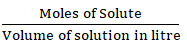(a) Molar mass of Co(NO)3.6H2O = 59 + 2 (14 + 3 × 16) + 6 × 18 = 291 g/mol
Therefore, Moles of Co(NO)3.6H2O = 30/291  mol
= 0.103 mol
Therefore, Molarity = 0.1o3/4.3 mol/lt = 0.023 M

(b) Number of moles present in 1000 mL of 0.5 M H2SO4 = 0.5 mol
Therefore, Number of moles present in 30 mL of 0.5 M H2SO4 =  0.5 × (30/1000)mol
= 0.015 mol
Therefore, Molarity = 0.015/0.5 L = 0.03 M

Que 4. Calculate the mass of urea (NH2CONH2) required in making 2.5 kg of 0.25 molal aqueous solution.
Ans 4. Moles of urea = 0.25 mole
Mass of solvent (water) = 1 kg = 1000 g
Molar mass of urea (NH2CONH2) = 2(1 × 14 + 2 × 1) + 1 × 12 + 1 × 16 = 60 g/mol
∴ Mass of urea in 1000 g of water = 0.25 mol × 60 g mol-1 = 15 g
Total mass of solution = 1000 + 15 g = 1015 g
2.5 kg means 2500 g
1015 g of solution contain urea = 15 g
1 g of solution contains urea = 15/1015
Therefore, 2500 g of solution contains Urea = 15 × 2500/1015
= 37g

Que 5. Calculate (a) molality (b) molarity and (c) mole fraction of Kl if the density of 20% (mass/mass) aqueous Kl is 1.202 g mL.
Ans 5. (a).  Calculation of molality of the solution
Weight of KI in 100 g of the solution = 20 g
Weight of water in the solution = 100 – 20 = 80 g
Molar mass of KI = 39 + 127 = 166 g mol-1
Therefore, the molality of the solution
= moles of KI/Mass of water in Kg  = (20/166)/ 0.08
= 1.506 m
= 1.51 m

(b) It is given that the density of the solution = 1.202 g mL-1
Mass / Density = Volume of 100 g solution
100g / 1.202 gml-1
= 83.19 mL
= 83.19 × 10-3 L
Therefore, molarity of the solution = (20/166) / 83.19 × 10-3 L
= 1.45 M
(c) Moles of KI
Moles of water = 80/18 = 4.44
Therefore, the mole fraction of KI = moles of KI/moles of KI + moles of water = 0.12/0.12 + 4.44
= 0.0263

NCERT Chemistry Class 12 Chapter 2 Intext Solutions

Que 6. H2S a toxic gas with a rotten egg-like smell is used for qualitative analysis. If the solubility of H2S in the water at STP is 0.195 m, calculate Henry’s law constant.
Ans 6. The solubility of H2S in the water at STP = 0.195 m
Mass of H2O = 1000 g
Number of moles of Water =  = 55.56 mol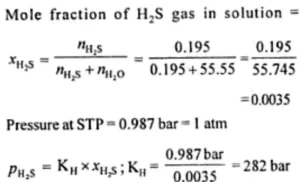Que 7. Henry’s law constant for CO2 in water is 1.67 × 108 Pa at 298 K. Calculate the quantity of CO2 in 500 mL of soda water when packed under 2.5 atm CO2 pressure at 298K.
Ans 7.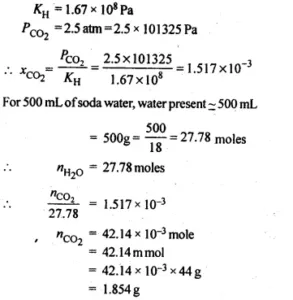NCERT Chemistry Class 12 Chapter 2 Intext Solutions

Que 8. The vapour pressures of pure liquids A and B are 450 and 700 mm Hg at 350 K respectively. Find out the composition of the liquid mixture if the total vapour pressure is 600 mm Hg. Also, find the composition of the vapour phase.
Ans 8. P0A = 450 mm of Hg
P0B = 700 mm of Hg
Ptotal​ = 600 mm of Hg
According to Raoult’s law:
PA = P0AXA      PB = P0BXB
We know XA + XB = 1
= XB = 1 – XA
Therefor PB = P0B (1-XA)
Therefore, total pressure, PTotal = PA + PB
PTotal = P0AXA + P0B (1-XA)
PTotal = P0AXA + P0B – P0B XA
PTotal = (P0A – P0B)XA + P0B
600 = (450 – 700) XA + 700
250 XA = 100
XA = 100/250 = 0.4
Therefore, XB = 1 – XA = 1 – 0.4 = 0.6
PA = P0AXA = 450 × 0.4 = 180 mm of Hg
PB = P0BXB = 700 × 0.6 = 420 mm of Hg
Now, in the vapour phase: Mole fraction of liquid A = PA / PA + PB
= 180 / 180 + 420
= 180/600 = 0.30
And, mole fraction of liquid B = 1 – 0.30 = 0.70

Que 9. The Vapour pressure of pure water at 298 K is 23.8 mm Hg. 50 g of urea (NH2CONH2) is dissolved in 850 g of water. Calculate the vapour pressure of water for this solution and its relative lowering.
Ans 9.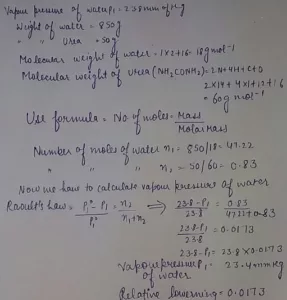NCERT Chemistry Class 12 Chapter 2 Intext Solutions

Que 10. The boiling point of water at 750 mm Hg is 99.63°C. How much sucrose is to be added to 500 g of water such that it boils at 100°C? The Molal elevation constant for water is 0.52 K kg mol-1.
Ans 10.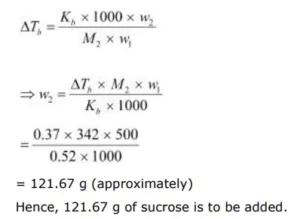Que 11. Calculate the mass of ascorbic acid (vitamin C, C6H8O6) to be dissolved in 75 g acetic acid to lower its melting point by 1.5°C, KF = 3.9 K kg mol-1.
Ans 11. Mass of acetic acid (w1) = 75 g
Molar mass of ascorbic acid (C6H8O6), M2­­ = 6 x 12 + 8 x 1 + 6 x 16 = 176 g mol-1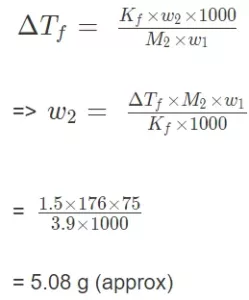Hence, the amount of ascorbic acid needed to be dissolved is 5.08 g.

Que 12. Calculate the osmotic pressure in pascals exerted by a solution prepared by dissolving 1.0 g of polymer of molar mass 185,000 in 450 mL of water at 37°C.
Ans 12. Volume of water (V) = 450 mL = 0.45 L
Temperature (T) = 37 + 273 = 310 K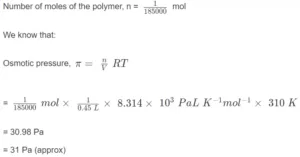NCERT Chemistry Class 12 Chapter 2 Intext Solutions